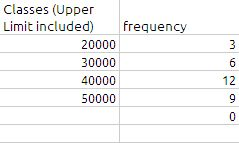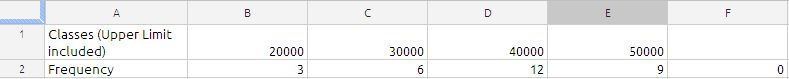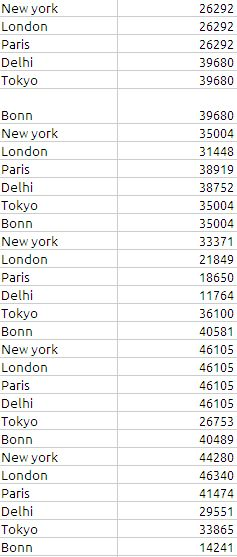# FREQUENCY

The FREQUENCY function returns an array of how often data values occur within a range of interval values.

FREQUENCY(data, classes)
data:  A collection containing the values to be evaluated. data is a collection containing number or date/time values. All values should be of the same type.

classes:  A collection containing the classes (interval values). interval-values is a collection containing number or date/time values. All values should be of the same type as the values in the data-values collection. Usage Notes

FREQUENCY determines the number of values in data that fall within each interval. The classes (interval array) is easiest to understand if it is arranged in ascending order. The first frequency will be the count of those values that are less than or equal to the lowest interval value. All other frequency values, except the last, will be the count of those values that are greater than the immediately lower interval value and less than or equal to the current interval value. The final frequency value will be the count of those data values that are greater than the largest interval value.

Note : Character sticks of Frequency function
1) Classes are the upper values for each category, and classes are the values to count.
2) FREQUENCY ignores empty cells and text.
3) FREQUENCY function always returns one more category than you give it so that it can  catch any values above the last category.
4) it always delivers a vertical array. So if we really need a horizontal array, we have to use TRANSPOSE function to convert the vertical array to a horizontal array.
5) If there are duplicate classes, the duplicates will get a count of zero (this aspect is utilized when you create formulas for unique counts).
6) since Frequency function happens to be array function google docs spread sheet, we do not need  to enter it with any special key combination.

Now lets see some example with Frequency function.
the formula in cell B2  is
=FREQUENCY(J1:J30,A2:A5)The value in cell B6 will come automatically even through there is no range is specified because the formula will include any value above 50000 in this range

If we want to table horizontal the formula will be modified as under:the formula in cell B3 is
=TRANSPOSE(FREQUENCY(J1:J30,B1:E1))

Since Frequency function ignore the duplicate. we can utilize this for counting unique value in the data range. The formula for counting the unique value in the range is

=ArrayFormula(sum(FREQUENCY(J1:J30,J1:J30)>0))
In the formula the secret is data and classes as same.

the result will be 21

Sample Data isAdvertisements